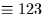Next: SLA_EVP - Earth Position & Velocity
Up: SUBPROGRAM SPECIFICATIONS
Previous: SLA_ETRMS - E-terms of Aberration

## SLA_EULER - Rotation Matrix from Euler Angles

ACTION:
Form a rotation matrix from the Euler angles - three successive rotations about specified Cartesian axes (single precision).

CALL:
CALL sla_EULER (ORDER, PHI, THETA, PSI, RMAT)

GIVEN:

 C*(*) ORDER specifies about which axes the rotations occur PHI R 1st rotation (radians) THETA R 2nd rotation (radians) PSI R 3rd rotation (radians)

RETURNED:

 R(3,3) RMAT rotation matrix

NOTES:
1.
A rotation is positive when the reference frame rotates anticlockwise as seen looking towards the origin from the positive region of the specified axis.
2.
The characters of ORDER define which axes the three successive rotations are about. A typical value is ZXZ', indicating that RMAT is to become the direction cosine matrix corresponding to rotations of the reference frame through PHI radians about the old z-axis, followed by THETA radians about the resulting x-axis, then PSI radians about the resulting z-axis. In detail:
• The axis names can be any of the following, in any order or combination: X, Y, Z, uppercase or lowercase, 1, 2, 3. Normal axis labelling/numbering conventions apply; the xyz () triad is right-handed. Thus, the ZXZ' example given above could be written zxz' or 313' (or even ZxZ' or 3xZ').
• ORDER is terminated by length or by the first unrecognized character.
• Fewer than three rotations are acceptable, in which case the later angle arguments are ignored.
3.
Zero rotations produces a unit RMAT.Next: SLA_EVP - Earth Position & Velocity
Up: SUBPROGRAM SPECIFICATIONS
Previous: SLA_ETRMS - E-terms of Aberration

SLALIB --- Positional Astronomy Library